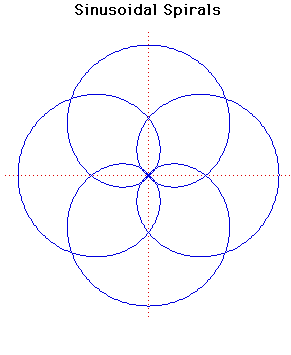# Sinusoidal SpiralsPolar equation:
rp = ap cos(pθ)

Click below to see one of the Associated curves.

Click THIS LINK to experiment interactively with this curve and its associated curves.

Sinusoidal spirals can have any rational number p in the formula above. Many standard curves occur as sinusoidal spirals.

If p = -1 we have a line.

If p = 1 we have a circle.

If p = 1/2 we have a cardioid.

If p = -1/2 we have a parabola.

If p = -2 we have a hyperbola.

If p = 2 we have a lemniscate of Bernoulli.

Sinusoidal spirals were first studied by Maclaurin.
They are not, of course, true spirals.

The pedal curve of sinusoidal spirals, when the pedal point is the pole, is another sinusoidal spiral.

The sinusoidal spiral rp = ap cos(pθ) inverts to rp = ap/cos(pθ) if the centre of inversion is taken at the pole.

JOC/EFR/BS January 1997